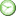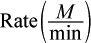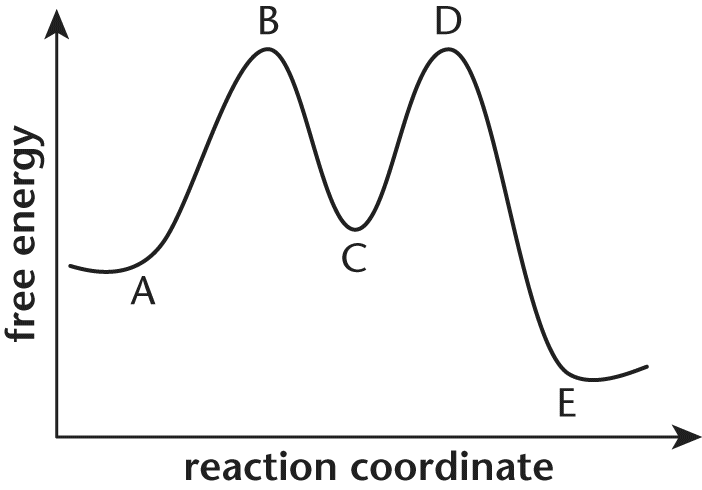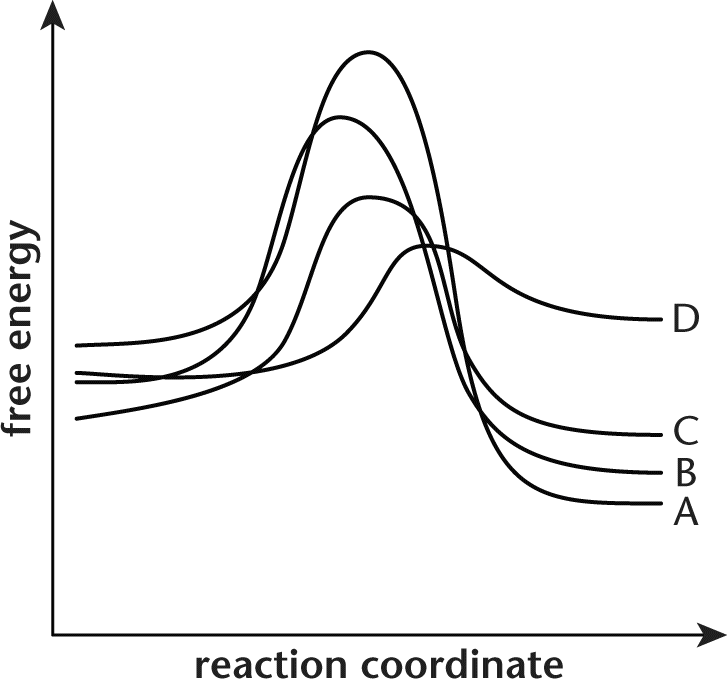# MCAT General Chemistry Practice Test 5: Chemical Kinetics

### Test Information15 questionsminutes

Take more free MCAT general chemistry practice tests available from maintests.com.

1. In a third-order reaction involving two reactants and two products, doubling the concentration of the first reactant causes the rate to increase by a factor of 2. What will happen to the rate of this reaction if the concentration of the second reactant is cut in half?

• A. It will increase by a factor of 2.
• B. It will increase by a factor of 4.
• C. It will decrease by a factor of 2.
• D. It will decrease by a factor of 4.

2. In a certain equilibrium process, the activation energy of the forward reaction is greater than the activation energy of the reverse reaction. This reaction is:

• A. endothermic.
• B. exothermic.
• C. spontaneous.
• D. nonspontaneous.

3. A reactant in a second-order reaction at a certain temperature is increased by a factor of 4. By how much is the rate of the reaction altered?

• A. It is unchanged.
• B. It is increased by a factor of 4.
• C. It is increased by a factor of 16.
• D. It cannot be determined from the information given.

4. The concentrations of all reactants in a zero-order reaction are increased twofold. What is the new rate of the reaction?

• A. It is unchanged.
• B. It is decreased by a factor of 2.
• C. It is increased by a factor of 2.
• D. It cannot be determined from the information given.

5. Which of the following experimental methods should NEVER affect the rate of a reaction?

• A. Placing an exothermic reaction in an ice bath.
• B. Increasing the pressure of a reactant in a closed container.
• C. Putting the reactants into an aqueous solution.
• D. Removing the product of an irreversible reaction.

6. What would increasing the concentration of reactants accomplish in a solution containing a saturated catalyst?

• A. It would increase the rate constant but not the reaction rate.
• B. It would decrease the rate constant but increase the reaction rate.
• C. It would increase the rate constant and increase the reaction rate.
• D. The reaction rate would be unaffected.

7. A certain chemical reaction has the following rate law:

rate = k[NO2][Br2]

Which of the following statements necessarily describe(s) the kinetics of this reaction?

I. The reaction is second-order.

II. The amount of NO2 consumed is equal to the amount of Br2 consumed.

III. The rate will not be affected by the addition of a compound other than NO2 and Br2.

• A. I only
• B. I and II only
• C. II and III only
• D. I, II, and III

8. The following data shown in the table were collected for the combustion of the theoretical compound XH4:

XH4 + 2 O2 → XO2 + 2 H2O

 Trial [XH4]initial (M) [O2]initial (M)1 0.6 0.6 12.4 2 0.6 2.4 49.9 3 1.2 2.4 198.3

What is the rate law for the reaction described here?

• A. rate = k[XH4][O2]
• B. rate = k[XH4][O2]2
• C. rate = k[XH4]2[O2]
• D. rate = k[XH4]2[O2]2

9. Which of the following best describes the purpose of a catalyst?

• A. Catalysts are used up in the reaction, increasing reaction efficiency.
• B. Catalysts increase the rate of the reaction by lowering the activation energy.
• C. Catalysts alter the thermodynamics of the reaction to facilitate the formation of products or reactants.
• D. Catalysts stabilize the transition state by bringing it to a higher energy.

10. If the rate law for a reaction is:

rate = k[A]0[B]2[C]1

What is the overall order of the reaction?

• A. 0
• B. 2
• C. 3
• D. 4

11. For question below, consider the following energy diagram shown below:The overall reaction depicted by this energy diagramis:

• A. endergonic, because point B is higher than point A.
• B. endergonic, because point C is higher than point A.
• C. exergonic, because point D is higher than point E.
• D. exergonic, because point A is higher than point E.

12. For question below, consider the following energy diagram shown below:Which process has the highest activation energy?

• A. The first step of the forward reaction
• B. The first step of the reverse reaction
• C. The second step of the forward reaction
• D. The second step of the reverse reaction

13. For question below, consider the following energy diagram shown below:Point C in this reaction profile refers to the:

• A. reactants.
• B. products.
• C. transition state.
• D. intermediates.

14. The following system obeys second-order kinetics.

 2 NO2 → NO3 + NO (slow) NO3 + CO → NO2 + CO2 (fast)

What is the rate law for this reaction?

• A. rate = k[NO2][CO]
• B. rate = k[NO2]2[CO]
• C. rate = k[NO2][NO3]
• D. rate = k[NO2]2

15. The potential energy diagram shown represents four different reactions.Assuming identical conditions, which of the reactions displayed on the energy diagram proceeds the fastest?

• A. A
• B. B
• C. C
• D. D

﻿